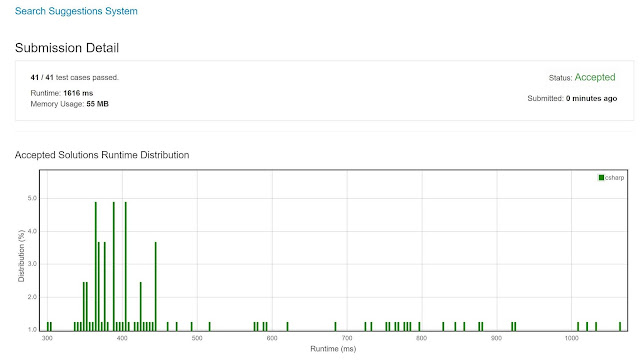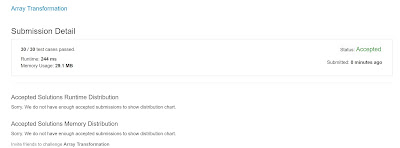## Posts

Showing posts from November, 2019

### Trie VariationHere is the problem:  https://leetcode.com/problems/search-suggestions-system/ 1268. Search Suggestions System Medium 63 39 Favorite Share Given an array of strings  products  and a string  searchWord . We want to design a system that suggests at most three product names from  products  after each character of  searchWord  is typed. Suggested products should have common prefix with the searchWord. If there are more than three products with a common prefix return the three lexicographically minimums products. Return  list of lists  of the suggested  products  after each character of  searchWord  is typed.  Example 1: Input: products = ["mobile","mouse","moneypot","monitor","mousepad"], searchWord = "mouse" Output: [ ["mobile","moneypot","monitor"], ["mobile","moneypot","monitor"], ["mouse","mousepad"], ["mouse","m

### Matrix in O(n)Here is the problem:  https://leetcode.com/problems/01-matrix/ 542. 01 Matrix Medium 919 92 Favorite Share Given a matrix consists of 0 and 1, find the distance of the nearest 0 for each cell. The distance between two adjacent cells is 1. Example 1: Input: [[0,0,0], [0,1,0], [0,0,0]] Output: [[0,0,0],  [0,1,0],  [0,0,0]] Example 2: Input: [[0,0,0], [0,1,0], [1,1,1]] Output: [[0,0,0], [0,1,0], [1,2,1]] Note: The number of elements of the given matrix will not exceed 10,000. There are at least one 0 in the given matrix. The cells are adjacent in only four directions: up, down, left and right. The idea will be to do 2-passes: from top to bottom (O(n)) and then from bottom to top (O(n)), using an extra matrix to keep track of the min distance. The first pass you check the min distance comparing with the previous row and the previous col. The second pass you keep track of the min between the current element and the next row, next col. Doing tha

### Two Sum Less Than K - An NLogN SolutionProblem is here:  https://leetcode.com/problems/two-sum-less-than-k/ Given an array  A  of integers and integer  K , return the maximum  S  such that there exists  i < j  with  A[i] + A[j] = S  and  S < K . If no  i, j  exist satisfying this equation, return -1. Example 1: Input: A = [34,23,1,24,75,33,54,8] , K = 60 Output: 58 Explanation: We can use 34 and 24 to sum 58 which is less than 60. Example 2: Input: A = [10,20,30] , K = 15 Output: -1 Explanation: In this case it's not possible to get a pair sum less that 15. Note: 1 <= A.length <= 100 1 <= A[i] <= 1000 1 <= K <= 2000 The approach is to sort the array and implement the "two pointers" technique. Have a left and right pointer. Notice that if the sum surpasses (or equal to) K, the only option is to move right down. If it is less than K, check if it is the max thus far and move the left up. Do this until the pointers cross each other. NLogN. Code is below, cheers, A

### Please use more memory, it is OK!Computer scientists and engineers: use more memory. It is fine. As a matter of fact, if you want to collaborate to ensure that Moore's Law (and the equivalent memory variant) remains true, then you actually have a duty to keep pushing memory (and CPU/GPU) to the next level! Memory these days is cheap - go on, and cache whatever you want. Search uses an absurd amount of caching and pre-computed data in memory. It is necessary. When you want to give the information back to the user in a blink of an eye, you have no choice but to do all the very heavy-lifting processing in the background, and "pre-cook" in memory a lot of what you want to serve the user. This is no cheating. It is technology. In-memory caching makes everyone's life easier - and faster. Here is a problem that exemplifies this concept:  https://leetcode.com/problems/range-sum-query-2d-immutable/ Given a 2D matrix  matrix , find the sum of the elements inside the rectangle defined by its upper lef

### Brace Expansion: a popular interview questionOne of the most popular interview questions - brace expansion, here it is:  https://leetcode.com/problems/brace-expansion/ A string  S  represents a list of words. Each letter in the word has 1 or more options.  If there is one option, the letter is represented as is.  If there is more than one option, then curly braces delimit the options.  For example,  "{a,b,c}"  represents options  ["a", "b", "c"] . For example,  "{a,b,c}d{e,f}"  represents the list  ["ade", "adf", "bde", "bdf", "cde", "cdf"] . Return all words that can be formed in this manner, in lexicographical order. Example 1: Input: "{a,b}c{d,e}f" Output: ["acdf","acef","bcdf","bcef"] Example 2: Input: "abcd" Output: ["abcd"] Note: 1 <= S.length <= 50 There are no nested curly brackets. All characters inside a pair

### Array Transformation using Bubble Sort TechniqueProblem is here:  https://leetcode.com/problems/array-transformation/ Given an initial array  arr , every day you produce a new array using the array of the previous day. On the  i -th day, you do the following operations on the array of day  i-1  to produce the array of day  i : If an element is smaller than both its left neighbor and its right neighbor, then this element is incremented. If an element is bigger than both its left neighbor and its right neighbor, then this element is decremented. The first and last elements never change. After some days, the array does not change. Return that final array. Example 1: Input: arr = [6,2,3,4] Output: [6,3,3,4] Explanation: On the first day, the array is changed from [6,2,3,4] to [6,3,3,4]. No more operations can be done to this array. Example 2: Input: arr = [1,6,3,4,3,5] Output: [1,4,4,4,4,5] Explanation: On the first day, the array is changed from [1,6,3,4,3,5] to [1,5,4,3,4,5]. On the second day, the array is c

### Palindrome PermutationHow can you check whether there exists a permutation P of a given string S such that P is a palindrome? This question has been asked here  https://leetcode.com/problems/palindrome-permutation/ . One way to solve it is to generate all the permutations of S and check for palindrome-ness for each one - that's exponential in time. But you can solve it in a linear pass. All you need to think about is the underlying structure of a palindrome. A palindrome can be created if and only if there exists at most one character in the string with an odd frequency count . With that rule in mind all you need to do is count the frequency of characters and perform the above check - that's it. Code is below, cheers, ACC. public class Solution {     public bool CanPermutePalindrome(string s)     {         if (String.IsNullOrEmpty(s)) return true;         Hashtable count = new Hashtable();         foreach (char c in s)         {             if (!count.ContainsKey(c)) count.Add(c, 0);